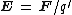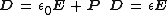electric field

(redirected from Electrostatic fields)
Also found in: Dictionary, Thesaurus, Medical.
Related to Electrostatic fields: Electric field strength

electric field

a field of force surrounding a charged particle within which another charged particle experiences a force

Electric field

A condition in space in the vicinity of an electrically charged body such that the forces due to the charge are detectable. An electric field (or electrostatic field) exists in a region if an electric charge at rest in the region experiences a force of electrical origin. Since an electric charge experiences a force if it is in the vicinity of a charged body, there is an electric field surrounding any charged body.

The electric field intensity (or field strength) E at a point in an electric field has a magnitude given by the quotient obtained when the force acting on a test charge q placed at that point is divided by the magnitude of the test charge q. Thus, it is force per unit charge. A test charge q is one whose magnitude is small enough so it does not alter the field in which it is placed. The direction of E at the point is the direction of the force F on a positive test charge placed at the point. Thus, E is a vector point function, since it has a definite magnitude and direction at every point in the field, and its defining equation is Eq. (1).

(1)Electric flux density or electric displacement D in a dielectric (insulating) material is related to E by either of the equivalent equations shown as Eqs. (2),
(2)where P is the polarization of the medium, and ε is the permittivity of the dielectric which is related to ε 0, by the equation ε = k ε 0, k being the relative dielectric constant of the dielectric. In empty space, D = ε 0 E .

In addition to electrostatic fields produced by separations of electric charges, an electric field is also produced by a changing magnetic field. See Electric charge, Electromagnetic induction, Potentials

Electric Field

a particular manifestation, along with the magnetic field, of an electromagnetic field that determines the effects upon an electric charge of a force that is independent of the velocity of the charge (see).

The concept of electric field was introduced into science by M. Faraday in the 1830’s. According to Faraday, each stationary charge creates an electric field in the surrounding space. The field of one charge acts upon another charge and vice versa, and an interaction between charges occurs (concept of short-range action). The principal quantitative characteristic of an electric field is its intensity E, defined as the ratio of the force F acting upon the charge to the magnitude of the charge q:E = F/q (seeFIELD INTENSITY, ELECTRIC). The electric field in a medium is characterized not only by its intensity but also by the electric induction vector (seeINDUCTION, ELECTRICAL AND MAGNETIC). The distribution of an electric field in space is visually represented by the intensity lines of force (seeLINES OF FORCE). The lines of force of a potential electric field that is created by electric charges originate on positive charges and terminate on negative charges. The lines of force of a rotational electric field, that is, an electric field created by a changing magnetic field, are closed curves.

The intensity of an electric field satisfies the principle of superposition, according to which for a given point in space, the intensity of the field E, created by several charges, is equal to the sum of the field intensities (E1, E2, E3, . . . .) of the individual charges: E = E1 + E2 + E3 + . . . . The superposition of fields follows from the linearity of Maxwell’s equations (seeMAXWELL’S EQUATIONS).

REFERENCES

Tamm I. E. Osnovy teorii elektrichestva, 9th ed. Moscow, 1976. Chapters 1 and 6.
Kalashnikov S. G. Elektrichestvo, 4th ed. Moscow, 1977. (Obshchii kura fiziki.) Chapters 2 and 13.

G. IA. MIAKISHEV

electric field

[i¦lek·trik ′fēld]
(electricity)
One of the fundamental fields in nature, causing a charged body to be attracted to or repelled by other charged bodies; associated with an electromagnetic wave or a changing magnetic field.
Specifically, the electric force per unit test charge.
References in periodicals archive ?
The binder and filler were mixed by applying a layer of the powder onto the filler surface in the electrostatic field with subsequent heating of the sample in order to melt and fix the polymer.
A dielectric circular cylinder with an eccentric circular cavity placed in a transverse electrostatic field, is analysed in .
Earlier experiments had shown two major problems: Electrons were being emitted from the cathode so as to form an uneven electric field that drew the ions toward the cathode instead of sending them past it; and beyond the cathode, electrostatic fields were forming that defocused the ion beam.
Since the two signal layers reside in each other's magnetic and electrostatic fields (between the same two reference planes), there is a lot of parasitic inductance and capacitance between the traces.
It shows the reliability of presented procedure for computation of nonhomogeneous electrostatic fields.
An electrostatic field meter may help identify the presence of electrostatic fields in the environment.
If the insulators in an environment are not producing electrostatic fields, why purchase ionizers to solve a non-existent problem?

Site: Follow: Share:
Open / Close